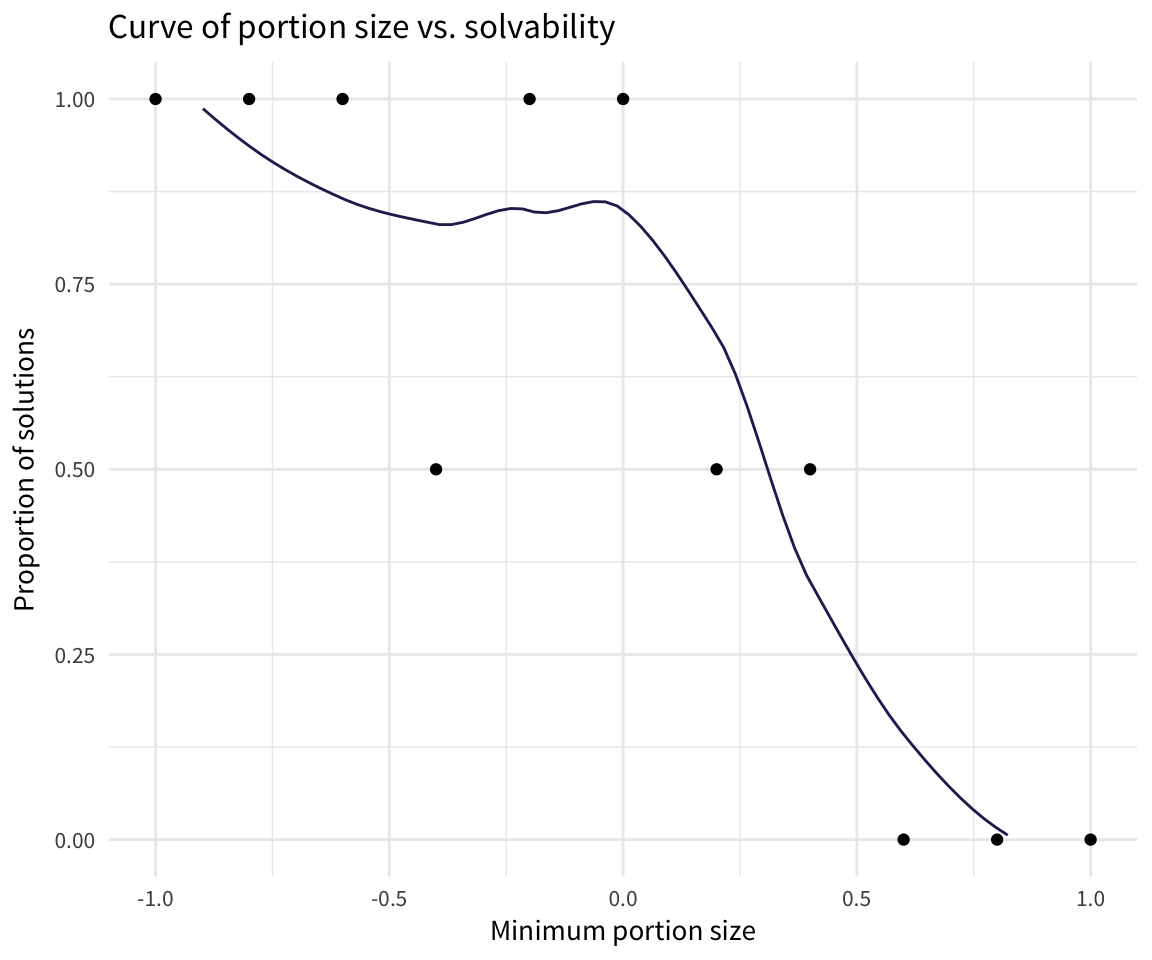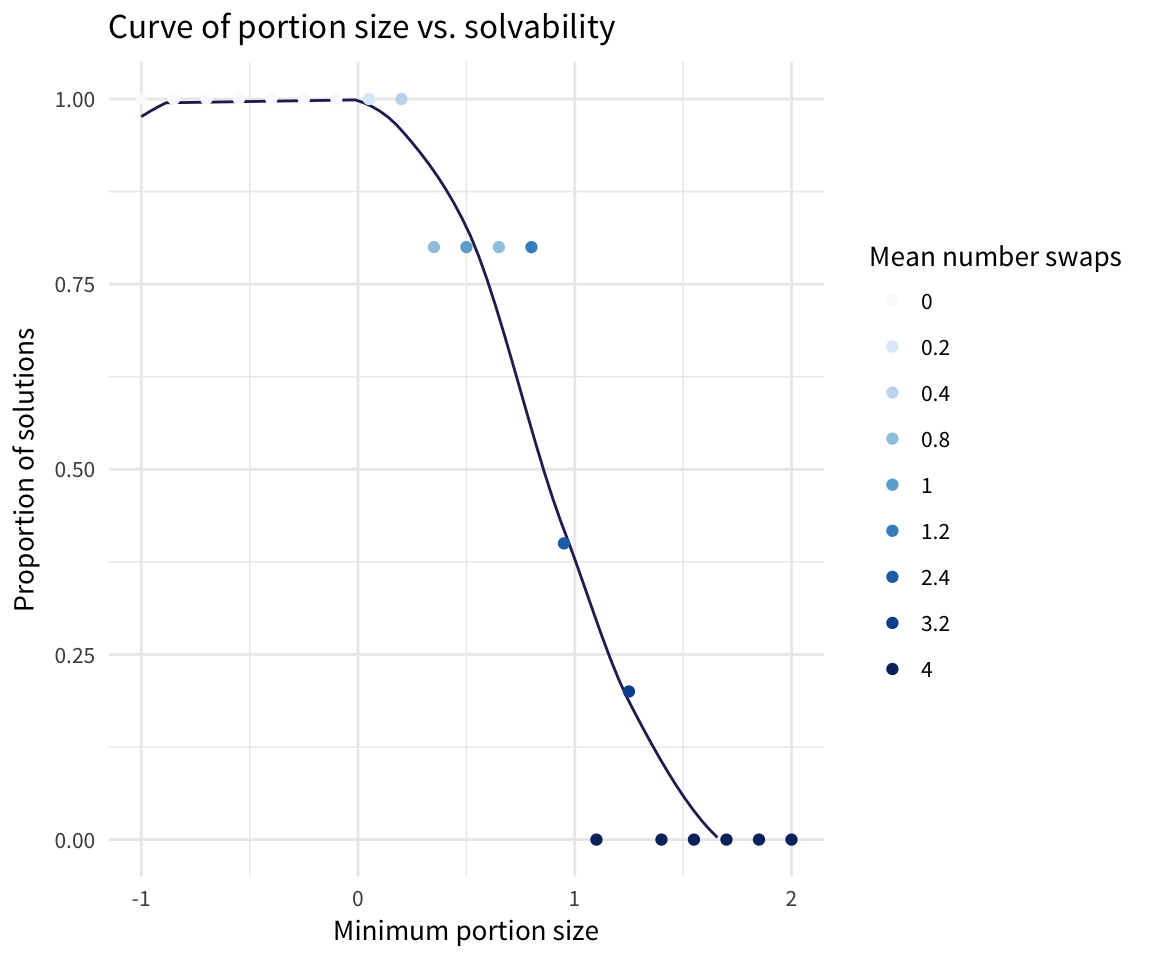# Food for Thought IV -- Simulation

Cool, so we’ve got a mechanism for creating and solving menus. But what portion of our menus are even solvable from the get-go? We’ll stipulate that solvable means solvable at a minimum portion size of 1 without doing any swapping. To answer that, I set about making a way to run a some simulations.

First, a helper funciton for just `pluck`ing the status portion of our `solve_it()` response telling us whether we solved the menu or not. The result of `get_status()` should always be either a 1 for unsolvable or 0 for solved.

``````get_status <- function(seed = NULL, min_food_amount = 0.5, verbose = TRUE) {
solve_it(min_food_amount = min_food_amount, verbose = verbose, only_full_servings = FALSE) %>%
purrr::pluck("status")
}``````

Now to the simulations: or a given minimum portion size, what proportion of a random number of simulated menus can we solve?

We’ll use `map_dbl` to get the status of each solution in our simulation. Then all we need to do is specify a minimum portion size for all menus to have and the number of simulations to run. We’ll shuffle the seed at which random menus are built for each simulation and then return a vector of whether each was solved or not.

``````simulate_menus <- function(n_sims = 10, min_food_amount = 0.5, verbose = FALSE) {

# Choose as many random seeds as we have simulations
seeds <- sample(1:n_sims, size = n_sims, replace = FALSE)

out <- seeds %>% map2_dbl(.y = min_food_amount, .f = get_status)
return(out)
}``````
``simulate_menus(verbose = FALSE)``
``## Cost is \$162.69.``
``## No optimal solution found :'(``
``## Cost is \$159.83.``
``## No optimal solution found :'(``
``## Cost is \$51.26.``
``## No optimal solution found :'(``
``## Cost is \$243.48.``
``## No optimal solution found :'(``
``## Cost is \$221.47.``
``## Optimal solution found :)``
``## Cost is \$97.09.``
``## No optimal solution found :'(``
``## Cost is \$84.67.``
``## Optimal solution found :)``
``## Cost is \$291.24.``
``## No optimal solution found :'(``
``## Cost is \$146.25.``
``## No optimal solution found :'(``
``## Cost is \$157.12.``
``## Optimal solution found :)``
``##   1 1 1 1 0 1 0 1 1 0``

Alright so that’s kinda useful for a single portion size, but what if we wanted to see how solvability varies as we change up portion size? Presumably as we decrease the lower bound for each food’s portion size we’ll give ourselves more flexibility and be able to solve a higher proportion of menus. But will the percent of menus that are solvable increase linearly as we decrease portion size?

#### Simulate Spectrum

I named this next function `simulate_spectrum()` because it allows us to take a lower and an upper bound of minimum portion sizes and see what happens at each point between those two intervals.

We specify the lower bound for the min portion size spectrum with `from` and the upper bound with `to`. How spaced out those points are and how many of them there are are set with `n_intervals` and `n_sims`; in other words, `n_intervals` is the number of chunks we want to split the spectrum of `from` to `to` into and `n_sims` is the number of times we want to repeat the simulation at each point.

Instead of a vector, this time we’ll return a dataframe in order to be able to match up the minimim portion size (`min_amount`, which we’re varying) with whether or not the menu was solvable.

``````simulate_spectrum <- function(n_intervals = 10, n_sims = 2, from = -1, to = 1,
min_food_amount = NULL, verbose = FALSE, ...) {

interval <- (to - from) / n_intervals
spectrum <- seq(from = from, to = to, by = interval) %>% rep(n_sims) %>% sort()

seeds <- sample(1:length(spectrum), size = length(spectrum), replace = FALSE)

out_status <- vector(length = length(spectrum))

for (i in seq_along(spectrum)) {
this_status <- get_status(seed = seeds[i], min_food_amount = spectrum[i], verbose = verbose)
if (!is.integer(this_status)) {
this_status <- integer(0)     # If we don't get an integer value back, make it NA
}
out_status[i] <- this_status
}

out <- tibble(min_amount = spectrum, status = out_status)

return(out)
}``````
``````status_spectrum <- simulate_spectrum()
status_spectrum %>% kable(format = "html")``````
min_amount status
-1.0 0
-1.0 0
-0.8 0
-0.8 0
-0.6 0
-0.6 0
-0.4 0
-0.4 1
-0.2 0
-0.2 0
0.0 0
0.0 0
0.2 1
0.2 0
0.4 1
0.4 0
0.6 1
0.6 1
0.8 1
0.8 1
1.0 1
1.0 1

#### Simulate Spectrum with Swapping

The next obvious question is, how many menus are solvable within a certain number of swaps?

`get_swap_status()` is analogous to `get_status()` from our vanilla simulator above. We specify a maximum number of allowed swaps. We build a random menu and count how many swaps it takes to solve it. If we can’t solve it within `max_n_swaps` swaps, we’ll give up. At the end, we return a tibble of the status and the number of swaps done for each food.

``````get_swap_status <- function(seed = NULL, min_food_amount = 0.5, max_n_swaps = 3, return_status = TRUE,
verbose = TRUE, ...) {
counter <- 0
this_solution <- build_menu(seed = seed) %>%
solve_it(min_food_amount = min_food_amount, verbose = verbose, only_full_servings = FALSE)

this_status <- this_solution %>% purrr::pluck("status")

while (counter < max_n_swaps & this_status == 1) {
this_solution <- this_menu %>% do_single_swap() %>%
solve_it(min_food_amount = min_food_amount, verbose = verbose, only_full_servings = FALSE)
this_status <- this_solution %>% purrr::pluck("status")

if (this_status == 0) {
message(paste0("Solution found in ", counter, " steps"))
if (return_status == TRUE) {
out <- list(status = this_status, n_swaps_done = counter) %>% as_tibble()
return(out)
} else {
}
}
counter <- counter + 1
}

message(paste0("No solution found in ", counter, " steps :/"))
out <- tibble(status = this_status, n_swaps_done = counter)
return(out)
}``````

Let’s test it.

``get_swap_status(seed = 12345)``
``## Cost is \$601.54.``
``## No optimal solution found :'(``
``## We've got a lot of CARROTS. 100 servings of them.``
``## Cost is \$508.88.``
``## No optimal solution found :'(``
``## Cost is \$551.81.``
``## No optimal solution found :'(``
``## Cost is \$365.01.``
``## No optimal solution found :'(``
``## No solution found in 3 steps :/``
``````## # A tibble: 1 x 2
##   status n_swaps_done
##    <int>        <dbl>
## 1      1           3.``````

So for this particular random menu that was created, we can see whether a solution was found and how many swaps we did to get there.

Now we can do the same for a spectrum of minimum portion sizes with a fixed max number of swaps we’re willing to do. Like we did with `simulate_spectrum()`, we split a minimum portion size (`from` to `to`) into `n_intervals` and do `n_sims` at each interval, recording the number of swaps it took to solve it. Our return tibble this time includes the minimum portion size we were allowed, the swap status (0 for good, 1 for bad), and the number of swaps we had to do

``````simulate_swap_spectrum <- function(n_intervals = 10, n_sims = 2, max_n_swaps = 3, from = -1, to = 1,
seed = NULL, verbose = FALSE, ...) {

interval <- (to - from) / n_intervals
spectrum <- seq(from = from, to = to, by = interval) %>% rep(n_sims) %>% sort()

if (!is.null(seed)) { set.seed(seed) }
seeds <- sample(1:length(spectrum), size = length(spectrum), replace = FALSE)

out_spectrum <- tibble(min_amount = spectrum)
out_status <- tibble(status = vector(length = length(spectrum)),
n_swaps_done = vector(length = length(spectrum)))

for (i in seq_along(spectrum)) {
this_status_df <- get_swap_status(seed = seeds[i], min_food_amount = spectrum[i], max_n_swaps = max_n_swaps, verbose = verbose)
if (!is.integer(this_status_df\$status)) {
this_status_df\$status <- integer(0)     # If we don't get an integer value back, make it NA
}
out_status[i, ] <- this_status_df
}

out <- bind_cols(out_spectrum, out_status)

return(out)
}``````
``````simmed_swaps <- simulate_swap_spectrum(n_intervals = 20, n_sims = 5, max_n_swaps = 4, seed = 2018,
from = -1, to = 2)
simmed_swaps %>% kable(format = "html")``````

#### Summarising a Spectrum

Let’s get some summary values out of our spectra. `summarise_status_spectrum()` allows us to summarise either a vanilla status spectrum or a swap spectrum. For a given portion size, we’ll find the proportion of those menus that are we were able to solve.

If we’ve allowed swapping, we’ll find the average number of swaps we did at a given portion size.

``````summarise_status_spectrum <- function(spec) {

# If this was a product of simulate_spectrum()
if (!"n_swaps_done" %in% names(spec)){
spec_summary <- spec %>%
group_by(min_amount) %>%
summarise(
sol_prop = mean(status)
)

# If this was a product of simulate_swap_spectrum()
} else {
spec_summary <- spec %>%
group_by(min_amount) %>%
summarise(
sol_prop = mean(status),
mean_n_swaps_done = mean(n_swaps_done)
)
}

return(spec_summary)
}``````

Let’s summarise our vanilla spectrum. `sol_prop` refers to the proportion of the recipes that we were able to solve.

``(status_spectrum_summary <- summarise_status_spectrum(status_spectrum))``
``````## # A tibble: 11 x 2
##    min_amount sol_prop
##         <dbl>    <dbl>
##  1     -1.00     0.
##  2     -0.800    0.
##  3     -0.600    0.
##  4     -0.400    0.500
##  5     -0.200    0.
##  6      0.       0.
##  7      0.200    0.500
##  8      0.400    0.500
##  9      0.600    1.00
## 10      0.800    1.00
## 11      1.00     1.00``````

Now the fun part: visualizing the curve of minimum allowed portion size per food compared to the proportion of menus that were solvable at that portion size.

``````ggplot() +
geom_smooth(data = status_spectrum, aes(min_amount, 1 - status),
se = FALSE,
colour = "#27265f",
size = 0.5) +
geom_point(data = status_spectrum_summary, aes(min_amount, 1 - sol_prop)) +
theme_minimal(base_family = "Source Sans Pro") +
ggtitle("Curve of portion size vs. solvability") +
labs(x = "Minimum portion size", y = "Proportion of solutions") +
ylim(0, 1) ``````So we don’t have a linear relationship between portion size and the porportion of menus that are solvable at that portion size.

We can do the same for our swap spectrum.

``simmed_swaps_summary <- summarise_status_spectrum(simmed_swaps)``

And plot our mininum portion size vs. whether we solved it or not.

``````ggplot() +
geom_smooth(data = simmed_swaps, aes(min_amount, 1 - status),
se = FALSE,
colour = "#27265f",
size = 0.5) +
geom_point(data = simmed_swaps_summary,
aes(min_amount, 1 - sol_prop,
colour = factor(mean_n_swaps_done))) +
# facet_wrap( ~ n_swaps_done) +
scale_colour_brewer(type = "seq", palette = 1) +
theme_minimal(base_family = "Source Sans Pro") +
ggtitle("Curve of portion size vs. solvability") +
labs(x = "Minimum portion size", y = "Proportion of solutions",
colour = "Mean number swaps") +
ylim(0, 1) ``````This makes intuitive sense that as we increase the required minimum portion size the number of swaps we need to do to get to a solvable menu increases, though I think I probably would have predicted a more linear relationship than we see here.

Next stop – web scraping! To get more realistic (read: paletable) menus, I’ll grab real recipes from Allrecipes.com and turn the unstructured text into something we can use to improve upon our existing methodology for generating and solving daily menus.